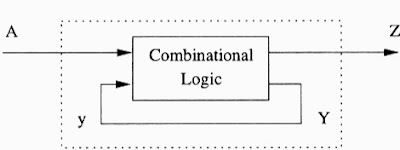# Sequential Circuits, Types (Synchronous and Asynchronous)

A logic circuit whose output at any instant of time depend not only on the present inputs but also on the past output is known as sequential circuit.

## Sequential Circuit

The logic circuits whose outputs at any instant of time depend not only on the present inputs but also on the past outputs are known as sequential circuits. In sequential circuit, outputs are fed back to the input side. Thus an output signal is a function of the present input signals and a sequence of the past input signals. The block diagram of sequential circuit is shown in Figure.Sequential Circuit

It consists of a combinational circuit to which memory elements are connected to form a feedback path. The storage elements are devices capable of storing binary information. The binary information stored in these elements at any given time defines the state of the sequential circuit at that time.

A sequential circuit is an interconnection of flip-flops and gates. The gates by themselves constitute a combinational circuit, but when included with the flip-flops, the overall circuit is classified as a sequential circuit. The sequential circuit consists of a combinational circuit and a number of clocked flip-flops.

As shown in the diagram, the combinational circuit block receives binary signals from external inputs and from the outputs of flip-flops. The output of flip-flops, in turn are applied to the combinational circuit inputs and determine the circuits behavior. This process demonstrates that the external outputs of a sequential circuit are function of both external inputs and the present state of flip-flops.

Thus a sequential circuit is specified by a time sequence of external input, external output and internal flip-flop binary states.

## Type of Sequential Circuits

Digital sequential circuits are divided into synchronous and asynchronous types. In synchronous sequential circuits, the state of the device changes only at discrete times in response to a clock signal. In asynchronous sequential circuits the state of the device can change at any time in response to changing inputs.

### (i) Synchronous Sequential Circuit

In synchronous sequential circuits, we do not depend upon unknown delays in the feedback path to give the memory function. Instead bi-stable memory elements in which we can store a '1' or a '0' are used. Information regarding the outputs is stored in the memories. The transfer of information from outputs to memories, is done only at preassigned manner. The contents of these memories are feedback as secondary inputs. A Block diagram of synchronous sequential circuit is shown in below Fig.Synchronous Sequential Circuits

Synchronous circuits are used in digital counters, shift registers, memory elements etc.

### (ii) Asynchronous Sequential Circuit

Asynchronous Sequential circuit, also called Event Driven Circuit does not have any clock to trigger change of state. State changes are triggered by change in input signal. In clock driven circuit all the memory elements change their states together. In asynchronous sequential circuit, the inputs and outputs do not change at preassigned time since the inherent delays are not rigidly controlled. This leads to known as races. If the input changes in a manner that warrants change in the state, it cannot do that immediately and wait till the next trigger comes. The block diagram of asynchronous sequential circuits is shown below Fig.Asynchronous Sequential Circuit

Asynchronous circuits are used in low power and high speed operations such as simple microprocessors, digital signal processing units and in communication systems for email applications, internet access and networking.

## Applications

• Sequential circuits are used as Shift registers.
• It are used in memory element (Flip flops).
• It is also used in Analog to digital and digital to analog converters.
• It is used as Counters.
• It is used as Clocks.